# Best Explanation of Apriori Algorithm for Association Rule Mining## Introduction

In this article, we will do an in-depth understanding of the Apriori algorithm for doing the association rule mining. We will first see what association rule mining really is and then understand some prerequisite concepts to the Apriori algorithm and finally do a deep dive into it with the help of an example. We will keep this article less theoretical and more practical with simple language for easy understanding for beginners.

## What is Association Rule Mining?

Association rule mining is a data mining technique to find hidden associations, frequent itemset patterns, and correlations within data. It is essentially a rule-based machine learning technique that generates rules by finding hidden patterns within the data.

A very popular application of association rule mining is Market Basket Analysis where POS sales data of supermarkets are mined to find hidden buying patterns of customers. Some buying habits are quite obvious like people who bought milk are very likely to buy bread as well.

But some patterns came as a surprise for e.g. beers and diapers were frequently purchased items because Dads who used to go to the market for purchasing diapers also used to buy beer!

Such hidden insights have helped supermarkets and stores to design their shelves accordingly. They place frequently purchased items within close proximity to prompt customers to purchase them together.

Alternatively, if the association is quite strong between the items then they can be placed on opposite ends of the store to prompt customers to walk all the way across with the hope they put more items in their basket on their way.

Now the online version of “customers who bought this item also bought” can be seen on e-commerce websites like Amazon, but instead of association rules they use more sophisticated recommendation techniques like collaborative filtering that are based on personalization.

## Prerequisite Concepts

### 1) k-Itemset

An itemset is just a collection or set of items. k-itemset is a collection of k items. For e.g. 2-itemset can be {egg,milk} or {milk,bread} etc. Similarily 3-itemset example can be {egg,milk,bread} etc.

### 2) Support Count

Support count is the number of times the itemsets appears out of all the transactions under consideration. It can also be expressed in percentage. A support count of  65% for {milk, bread} means that out both milk and bread appeared 65 times in the overall transaction of 100.

Mathematically support can also be denoted as –

support(A=>B) = P(AUB)

This means that support of the rule “A and B occur together” is equal to the probability of A union B.

### 3) Confidence

For a proposed rule A=>B, confidence is the number of times B appeared in the transaction given that A is already present. A confidence of 80% for milk=>bread implied that 80% of the customers who purchased milk also purchased bread.

Mathematically, confidence can be denoted as the probability of B given A –

confidence(A=>B) = P(B|A)

Further, confidence can be denoted in terms of support as below –

confidence(A=>B) = P(B|A) = $$\frac{support(AUB)}{support(A)}$$

### 4) Frequent Itemset

An itemset is said to be a frequent itemset when it meets a minimum support count. For example, if the minimum support count is 70% and the support count of 2-itemset {milk, cheese} is 60% then this is not a frequent itemset.

The support count is something that has to be decided by a domain expert or SME.

## Association Rule Algorithm

The association rules are derived with the below algorithm –

1. First, calculate all the frequent itemset from the list of transactions
2. For each frequent itemset L, we first generate all non-empty subsets of the itemset L.
3. Now for each subset s derived above, we create all candidate rules as S => (L-S)
4. Candidate rule S=>(L-S) is an associate rule only if it meets minimum confidence

You may not have realized this but finding frequent itemsets from hundreds and thousands of transactions that may have hundreds of items is not an easy task. We need a smart technique to calculate frequent itemsets efficiently and Apriori algorithm is one such technique.

## What is Apriori Algorithm?

Apriori algorithm is a very popular technique for mining frequent itemset that was proposed in 1994 by R. Agrawal and R. Srikant.

In the Apriori algorithm, frequent k-itemsets are iteratively created for k=1,2,3, and so on… such that k-itemset is created by using prior knowledge of (k-1) itemset. For e.g 3-itemset is generated with prior information of 2-itemset, 4-itemset is created with prior knowledge of 3-itemset and so on.

Because it uses prior knowledge of (k-1)itemset for creating k-itemset, it is named as Apriori algorithm.

## How Apriori Algorithm Works?

Before we see how Apriori algorithm works we need to understand two concepts of Joining and Pruning that are very important steps for Apriori algorithm.

### Joining

In the join step, to generate k-itemsets, (k-1) itemset is joined with itself without duplication. For example. For example, 2-itemsets {A,B} and {B,C} can be joined to create 3-itemset {A,B,C}

### Pruning

It should be noted that for finding support count of k-itemset we have to do a full scan of the database each time which is a costly operation. To improve the performance of the Apriori algorithm we use of Apriori property to reduce the search space of itemsets. Let us see what this property says.

#### Apriori Property

“All non-empty subsets of a frequent itemset must also be frequent.”

To explain this property let us consider 3-itemset {a,b,c} then Apriori property states that all its non empty subsets {a},{b},{c},{a,b},{b,a},{a,c} should also be frequent itemsets, i.e. they need to satisfy the minimum support count.

This property helps to discard or prune candidate itemsets in the Apriori algorithm that contain subsets that are non-frequent. We will understand this in more detail in the example.

Now let us see how Apriori algorithm works.

### Apriori Algorithm

1) Generate 1-Itemset

2)  Start Loop with K=2

3)      Generate candidate K-itemsets by joining (k-1) itemset

4)      Apply Prunning on the candidate k-itemsets

5)      If the k-itemset becomes empty after pruning then Stop Else continue to next step

6)      For each Candidate K-itemset Start Loop

7)                     Calculate Support Count of current K-itemset

8)                     If Support Count < Threshold then discard the current K-itemset

9)                     Else keep the current K-itemset

10)        End Loop

11)       K=K+1

12)  End Loop

The below diagram will give you a more clear understanding of the algorithm.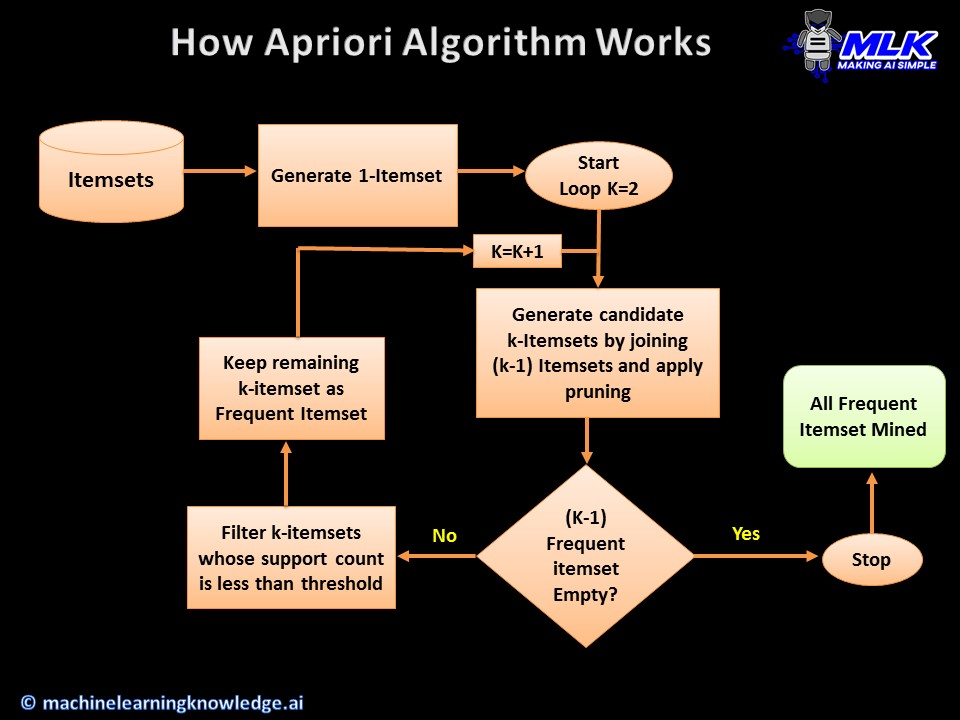## Apriori Algorithm Example

Let us now see an example of Apriori algorithm example for associating rule mining for better clarity.

For this purpose let us consider an example dataset of various transactions having multiple items.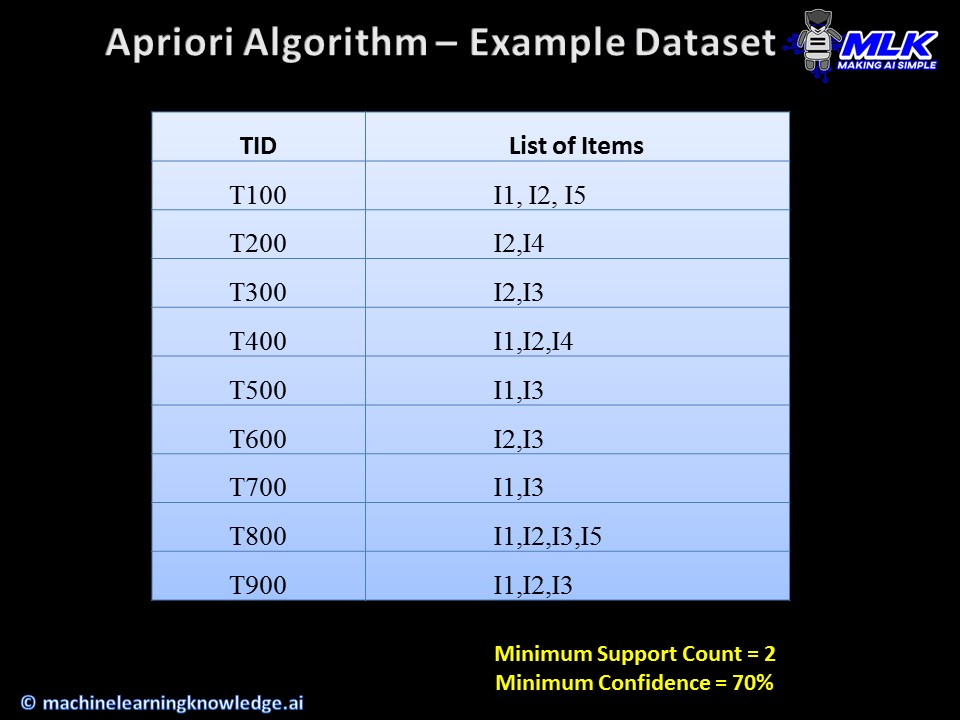### Iteration – 1

In the first iteration, things are quite straightforward. We first create candidate 1-itemsets C1 from the given dataset along with the support count of each. Here all of them satisfy the minimum support count of 2 and hence they all qualify as frequent 1-itemsets L1.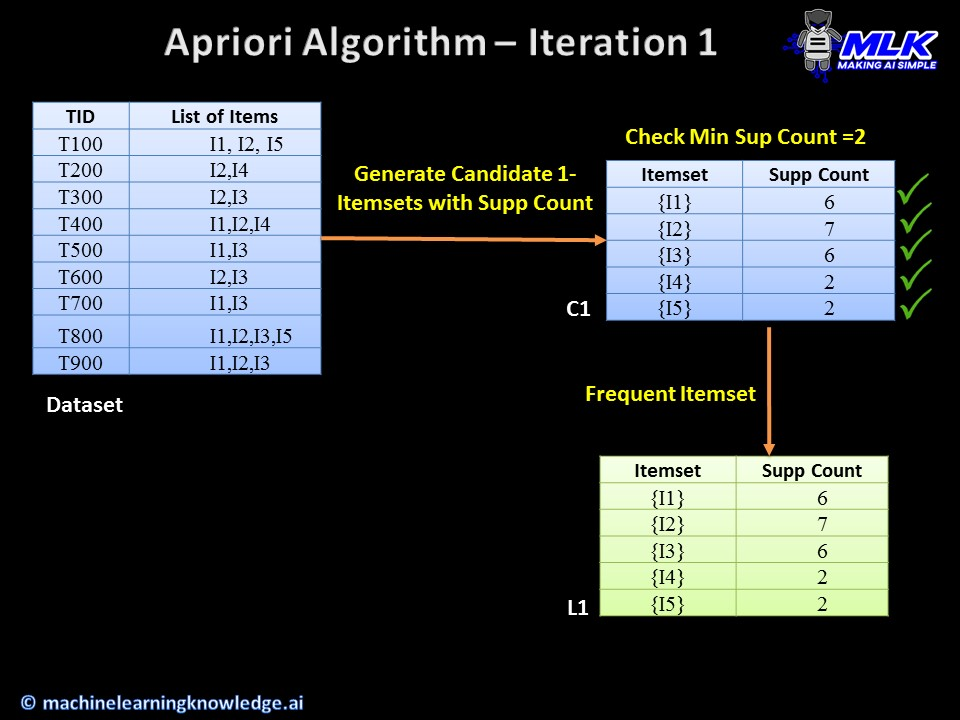### Iteration – 2

In the 2nd iteration, we create candidate 2-itemsets C2 by joining L1XL1. In the candidate itemsets, we don’t have anything to prune as all of its subsets are present in frequent 1-itemset L1.

We next calculate the minimum support count for C2 from the dataset and remove those datasets that do not meet the threshold. Finally, we are left with frequent 2-itemsets L2.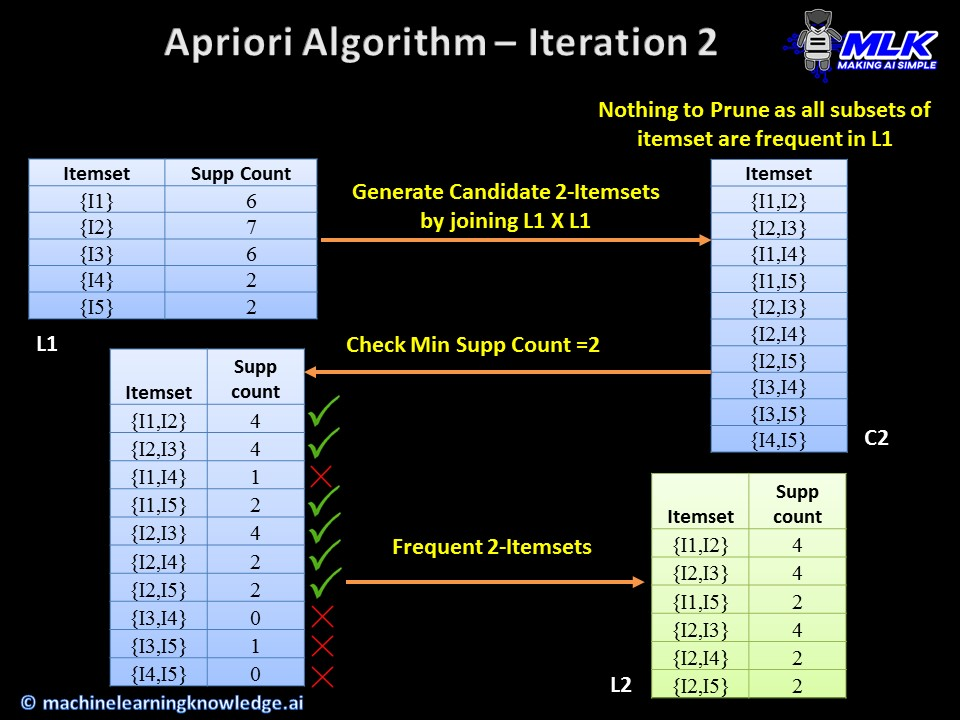### Iteration – 3

In the 3rd iteration, we create candidate 3-itemsets C3 by joining L2XL2. Here we prune some itemsets as their subset (highlighted in red) does not appear in frequent 2-itemset L2 This is as per Apriori property we discussed had discussed in the above section.

After pruning, we calculate the support count of the remaining candidate itemset and they all satisfy the minimum threshold of 2.

We are finally left with the frequent 3-itemset L3.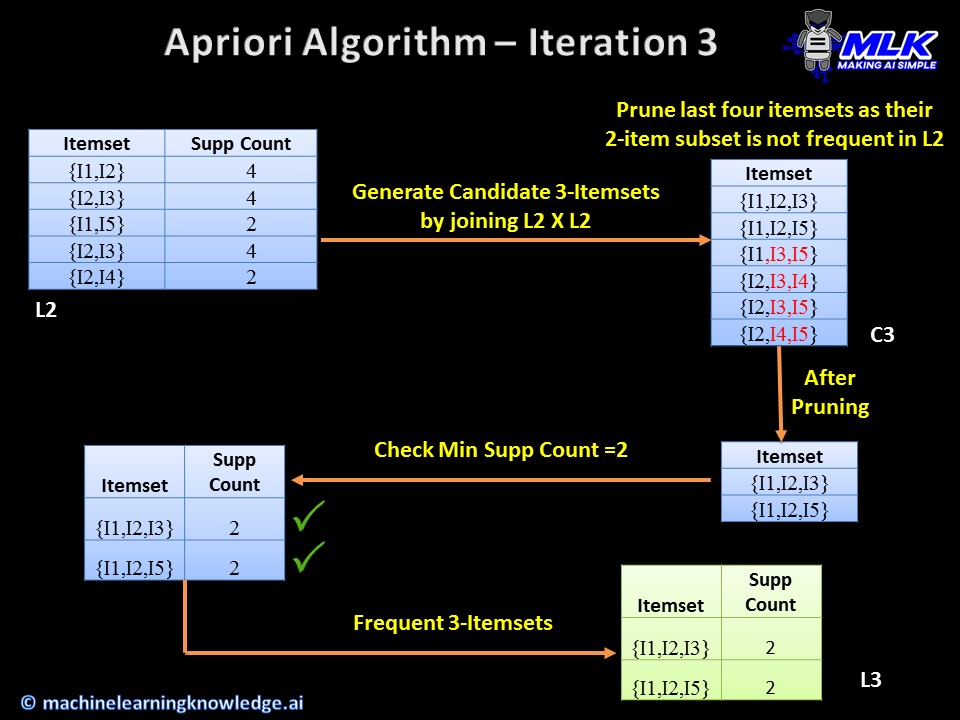### Iteration – 4

In the 4th iteration, we create the candidate 4-itemsets C4 by joining L3XL3. But we have to prune the only candidate 4-itemset since its subset (highlighted in red) does not appear in the frequent 3-itemset L3.

Thus we are left with an empty set after pruning and we stop the Apriori algorithm here having generated the frequent itemsets.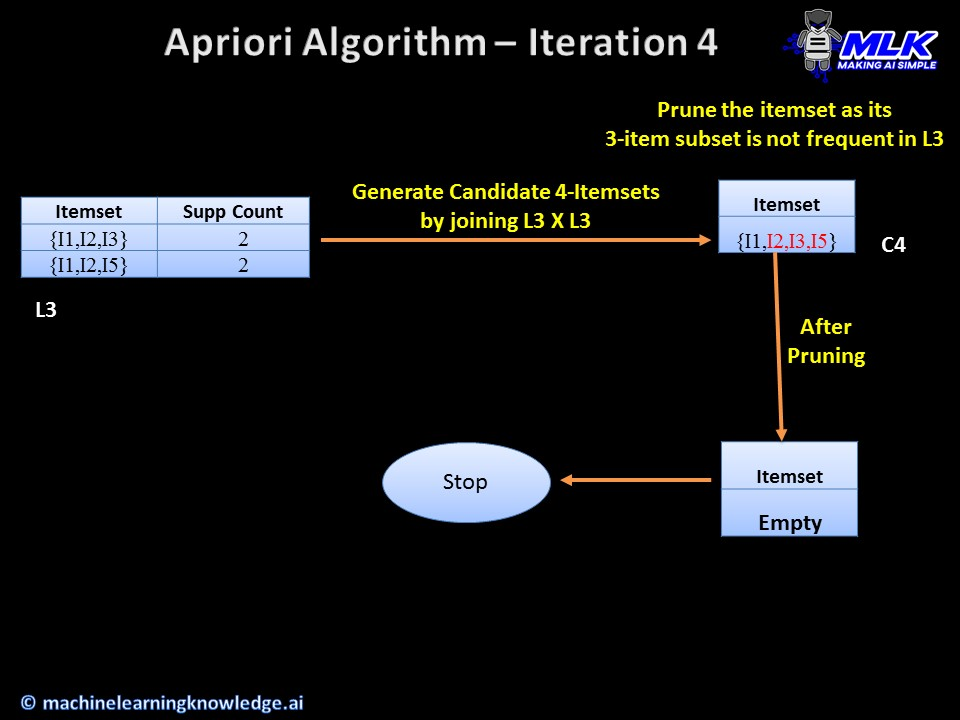## Example of Generating Association Rule

Now that we have seen an example of Apriori algorithm, let us now see how we can us the frequent itemset generated in the above example to generate the association rule.

Let us consider frequent 3-itemset {I1,I2,I5} for generating association rule. Following the algorithm of association rule, we generate non-empty subsets of {I1,I2,I5} and create the rules as illustrated in the diagram below.

We then calculate the confidence of each rule, and if we assume that minimum confidence to be 80% then only three rules can be considered as association rules.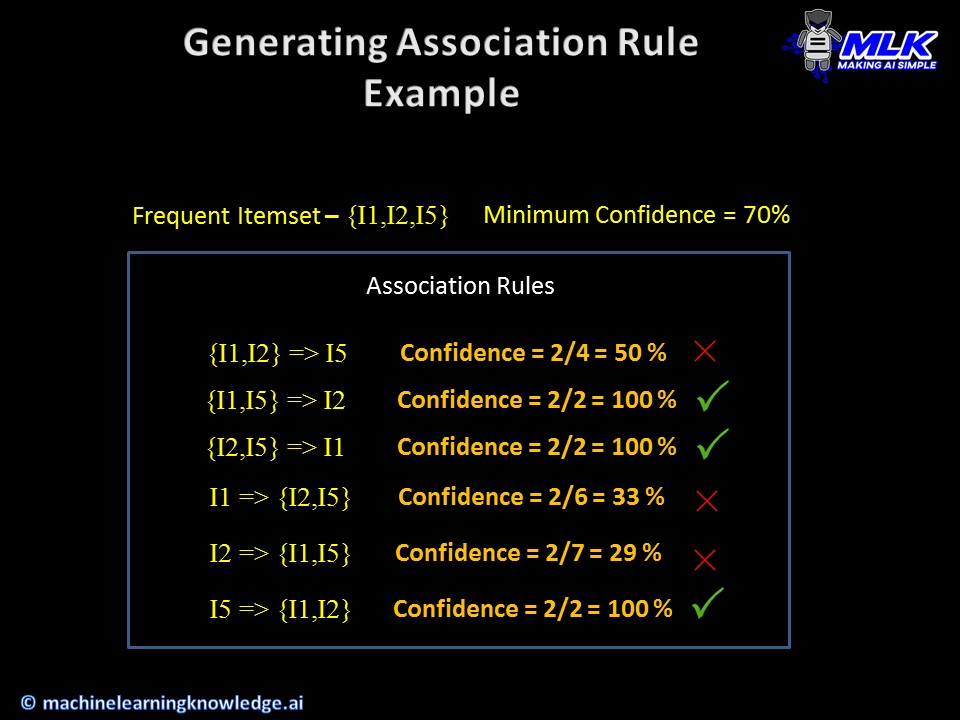## Conclusion

In this article, we understood Apriori algorithm for doing the association rule mining. We first saw what association rule mining really is and then understood some prerequisite concepts to the Apriori algorithm. Finally, we went through an example for doing association rule mining with Apriori algorithm.